Section B

# Ellipsoid Modeling Method for Coding of Face Depth Picture

Dong-jin Park1, Soon-kak Kwon1,*
1Dept. of Computer Software Engineering, Dongeui University, Busan, Rep. of Korea
*Corresponding Author : Soon-kak Kwon, (47340) Eomgang-ro 176, Busanjin-gu, Busan, Korea, +82-51-890-1727, skkwon@deu.ac.kr

© Copyright 2019 Korea Multimedia Society. This is an Open-Access article distributed under the terms of the Creative Commons Attribution Non-Commercial License (http://creativecommons.org/licenses/by-nc/4.0/) which permits unrestricted non-commercial use, distribution, and reproduction in any medium, provided the original work is properly cited.

Received: Nov 12, 2019; Revised: Dec 19, 2019; Accepted: Dec 23, 2019

Published Online: Dec 31, 2019

## Abstract

In this paper, we propose an ellipsoid modeling method for coding of a face depth picture. The ellipsoid modeling is firstly based on a point of a nose tip which is defined as the lowest value of the depth in the picture. The proposed ellipsoid representation is simplified through a difference of depth values between in the nose tip and in left or right boundary point of the face. Parameters of the ellipsoid are calculated through coordinates and depth values to minimize differences from the actual depth pixels. A picture is predicted by the modeled ellipsoid for coding of the face depth picture. In simulation results, an average MSEs between the face depth picture and the predicted picture is measured as 20.3.

Keywords: Ellipsoid Modeling; Depth Picture; Face Recognition

## I. INTRODUCTION

Recently the face recognition technology for identity recognition is increasing. In particular, the face recognition methods based on the color image [1-5] have been researched. However, the face recognition method based on color image is vulnerable to false authentication attempts using photographs. To solve this problem, the face recognition methods based on the depth image whose pixels have distances from the camera has been proposed [6-7]. This method recognizes the face by using LBP (Local Binary Patterns) of a depth picture with a face. This method improved a face recognition accuracy in dark environment compared to methods based on color image, and false authentication attempt can be prevented.

The processes of identity recognition system have a face capturing step, a face detection step, and a face recognition step. In the face detection step, the existence of a face in the image is determined and an area of a face is detected. In the face recognition step, features of the face are extracted and compared to stored face features. When a face picture is captured by an embedded device, it is inefficient to perform the face recognition step on the same device because its processor does not perform well. Therefore, the step of face recognition that are extracting and comparing face features is efficient to be processed separately in a high performance device. In order to implement the real time system for the face recognition, it is important to improve the speed of face picture transmission between an embedded device for capturing depth pictures and a device for extracting facial features. The improvement of the transmission speed can be achieved by efficiently compressing the depth pictures including the face.

Several methods for compressing depth video through conventional video coding standards for color video have been studied [8-13]. However, the coding schemes designed for color video are not directly applicable to the depth video since the number of bits for a depth pixel is higher than 8 bits of a color pixel.

The depth picture can be regarded as representation of surfaces. Therefore, the depth picture can be predicted by plane modeling  or spherical surface . In order to predict a face depth picture, the ellipsoid modeling is proposed because the shape of the face is close to an ellipsoid.

The human face is similar to an ellipsoid. Therefore, the depth picture that is captured the face is also similar to the ellipsoid surface. An amount of transmission information of the face depth picture can be reduced by predicting the picture through modeled the ellipsoid. In this paper, we propose a method of ellipsoid modeling for the face depth picture. The nearest ellipsoid is modeled using depth pixels in the face picture. Depth pixel values are predicted through the modeled ellipsoid.

## II. ELLIPSOID MODELING FOR FACE DEPTH PICTURE

2.1 Transformation of image coordinates to 3D coordinates through depth value

A camera is a kind of device used to translate coordinates. A point in the real world is projected into a pixel on a plane, which is an image captured by the camera. The pinhole camera model assumes that a point in real world passes through the pinhole and is projected into a temporary image plane. In the actual pinhole camera, the projected image plane is located behind the pinhole plane back so the image is overturned. However, the pinhole camera model assumes that the image plane is placed in the front of the pinhole. A point in 3D camera coordinate system, whose origin is set to the camera and direction of z-axis is set to the optical axis of the camera, projected onto a point in the image plane as shown in Fig. 1. In this case, x- and y-coordinates of the projected point are defined as the coordinates of its pixel. The relationship between the 3D camera coordinates and the image coordinates as follows:

$\begin{array}{l}z:f=x:X\to x=\frac{Xz}{f}\hfill \\ z:f=y:Y\to y=\frac{Yz}{f},\hfill \end{array}$
(1)

where f means a focal length, which is a distance between the image plane and the camera. Therefore, the image coordinates (X, Y) of a pixel with a depth value d(X, Y) are transformed into 3D camera coordinates (x, y, z) as follows:

$x=\frac{X}{f}d\left(X,Y\right),y=\frac{Y}{f}d\left(X,Y\right),z=d\left(X,Y\right).$
(2)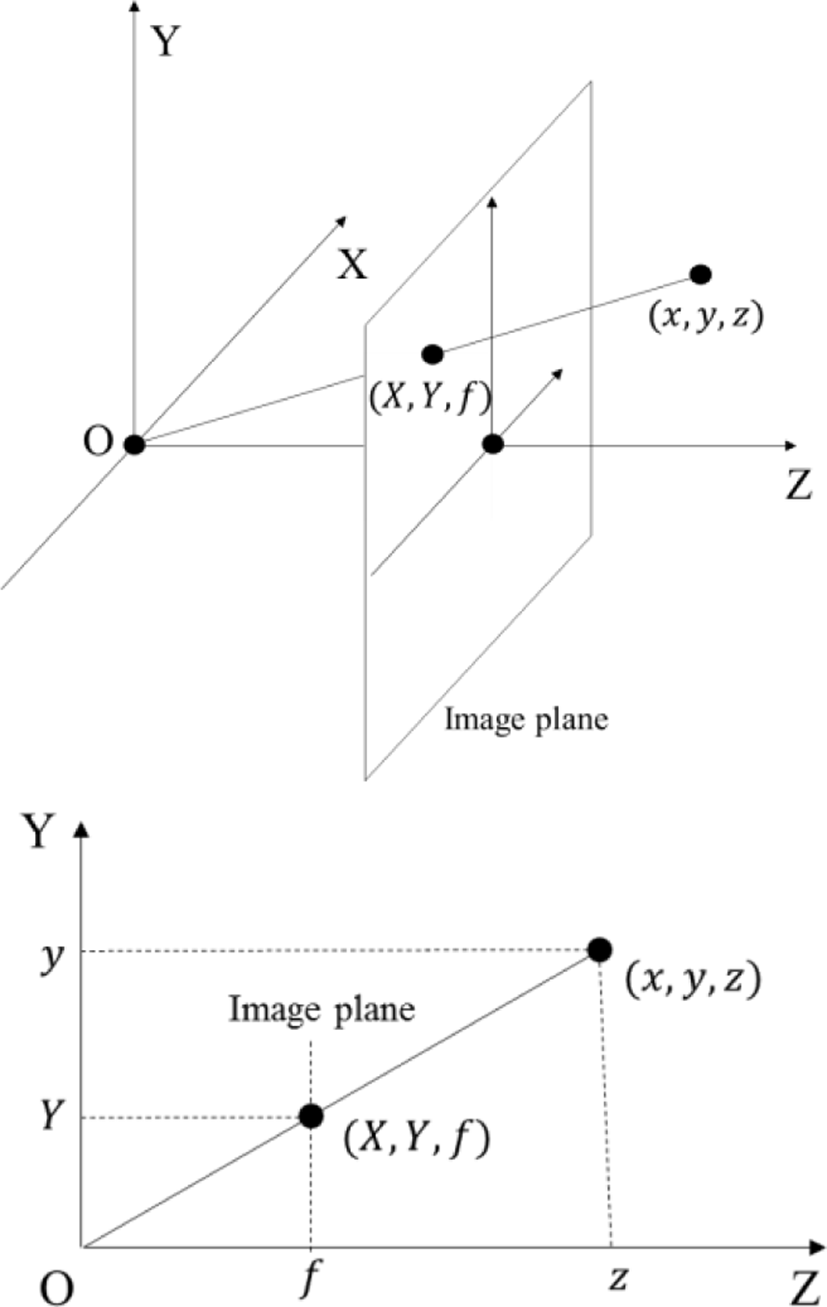Fig. 1. Relationship between camera and image coordinates in pinhole camera model.
2.2 Detection of nose tip from face depth picture

A human face is similar to an ellipsoid. A nose is close to the center of the face. Therefore, it is important to detect the nose in a face depth picture for the ellipsoid modeling.

In the face depth picture, a nose tip is usually the closest distance from the camera so the depth of the nose tip has a minimum value when the face is aligned with the direction of the camera capturing as shown in Fig. 2 (a). However, the other point such as jaw can have a minimum value in the depth picture as shown in Fig. 2 (b). In order to solve this problem, points having a minimum depth value in local area instead of whole picture area are found as candidates of the nose tip.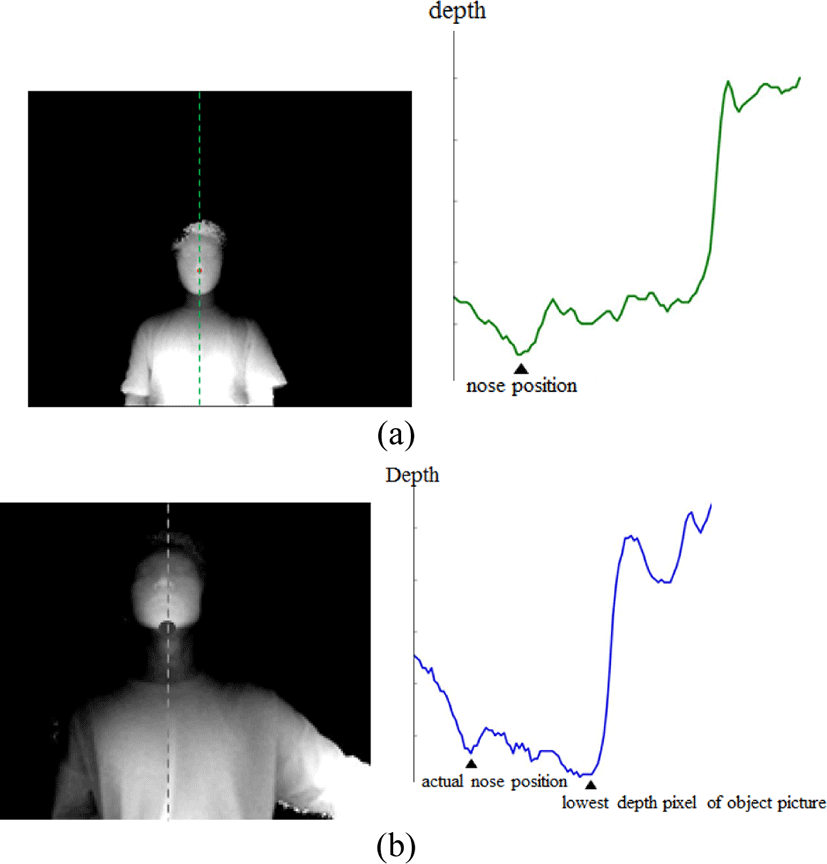Fig. 2. Depth value of nose tip according to face poses. (a) in case of frontal capturing, and (b) in case of capturing from below.

The depth values decrease continuously as it gets closer to the nose tip. Therefore, candidates of nose tip can be found by searching pixels each which has the smallest depth value in a local area. Each pixel is searched vertically and horizontally to the region performed in order to find a pixel whose N consecutive pixels in the left and right directions become larger depth value than the preceding pixel through following equation:

$\begin{array}{l}{p}_{i}<{p}_{i+1}<{p}_{i+2}<\cdots <{p}_{i+N}\hfill \\ {p}_{i}<{p}_{i-1}<{p}_{i-2}<\cdots <{p}_{i-N}.\hfill \end{array}$
(3)

where pi means a searched pixel and pi±k means a kth pixel from pi in the vertical or the horizontal directions.

In order to detect actual nose tip, it is necessary to compare the neighboring pixels of the candidates. Each candidate is compared with depth values of surrounding pixels. Each surrounding pixel whose distance is M (M > N) pixels are compared with the candidate point as shown in Fig. 3. If a depth of a candidate point is less than every surrounding pixel, the candidate point is determined as the actual nose tip as shown in Fig. 4.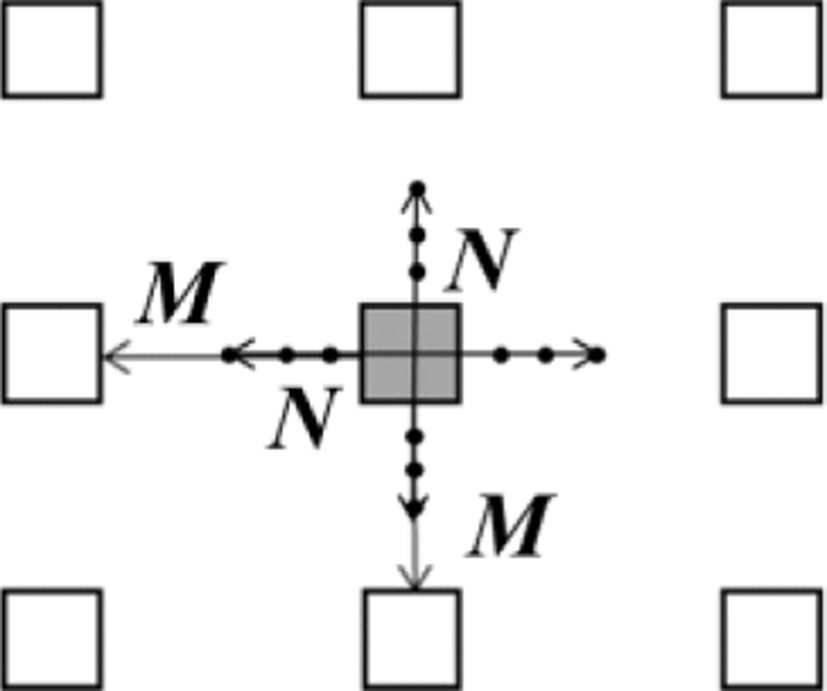Fig. 3. Comparison of depth value with surrounding pixels.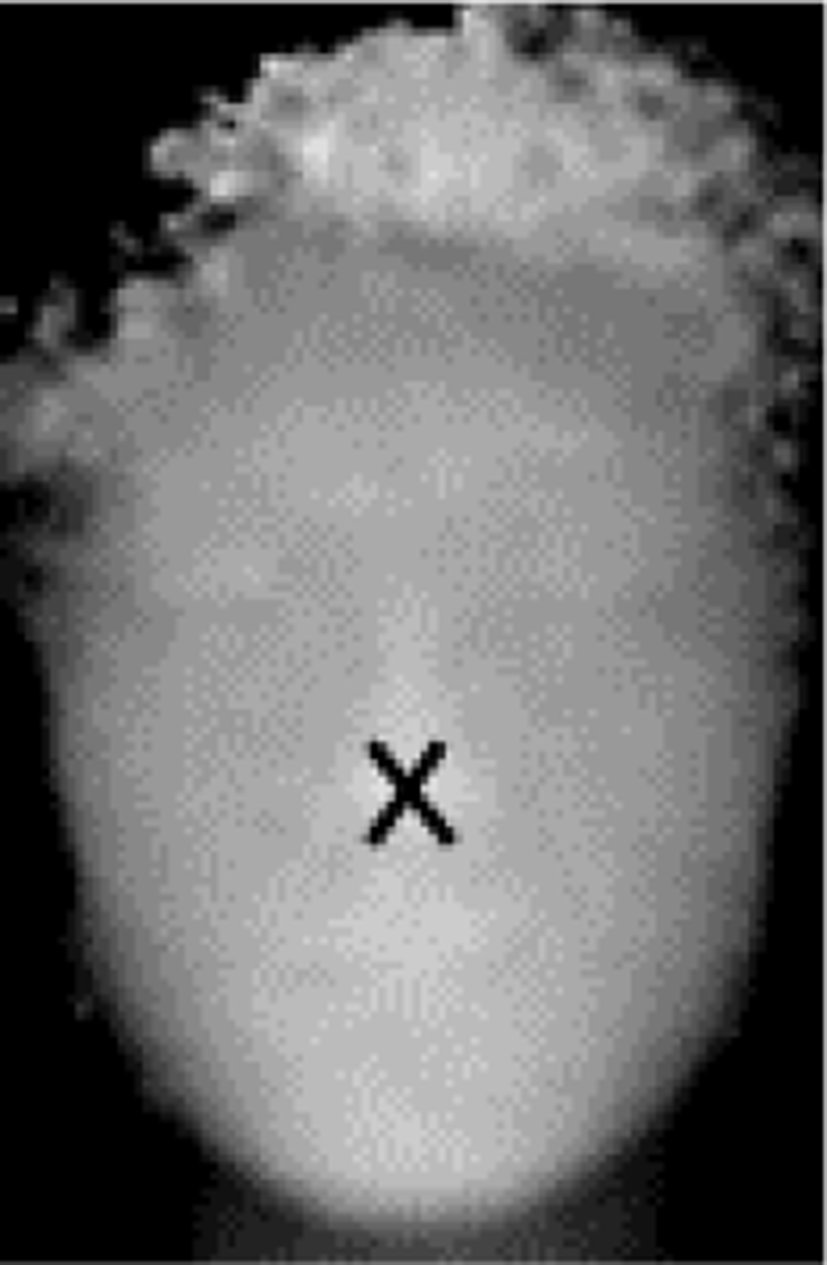Fig. 4. Found nose point from face depth picture.
2.3 Ellipsoid Modeling for Face Depth Picture

A representation equation of an ellipsoid in camera coordinate system is as follows:

${x}^{2}/{a}^{2}+{y}^{2}/{b}^{2}+{\left(z-c\right)}^{2}/{c}^{2}=1,$
(4)

where a, b, c mean parameters of the ellipsoid and c means radius of the ellipsoid for the z-axis. Therefore, c can be approximated by the distance δ between depth values of the nose tip in the face and of a face boundary point. In this paper, the depth value of the face boundary point is set to a depth value of a left or right boundary point relative to the nose point. Therefore, δ is a difference between the depth values of nose and boundary points. Eq. (4) is modified to a representation for d as follows:

$\begin{array}{l}\left(\frac{1}{{\delta }^{2}}\right)d{\left(X,Y\right)}^{2}+\frac{2}{\delta }d\left(X,Y\right)+\frac{{x}^{2}}{{\left(fa\right)}^{2}}+\frac{{y}^{2}}{{\left(fb\right)}^{2}}=0,\\ \delta =\mathrm{max}\left(|{d}_{nose}-{d}_{l}|,|{d}_{nose}-{d}_{r}|\right).\end{array}$
(5)

where dnose, dl, dr mean depth values of nose, left boundary point, and right bound point, respectively.

The depth value of the pixel located at (X, Y) with respect to the parameters a and b of the ellipsoid is solution of (5). At this time, 2 solutions of quadratic equations can be found as shown in Fig. 5. This means that 2 depth values can be predicted in the modeled ellipsoid. However, a surface of the face corresponds to the inner surface of the ellipsoid. Therefore, only the depth value of the inner surface is needed. The depth value can be predicted as follows:

$\overline{d}\left(X,Y\right)=-c\left(1-\sqrt{1-4\left({X}^{2}/{a}^{2}+{Y}^{2}/{b}^{2}\right)}\right).$
(6)

where (X,Y) means a prediction depth value at position (X, Y).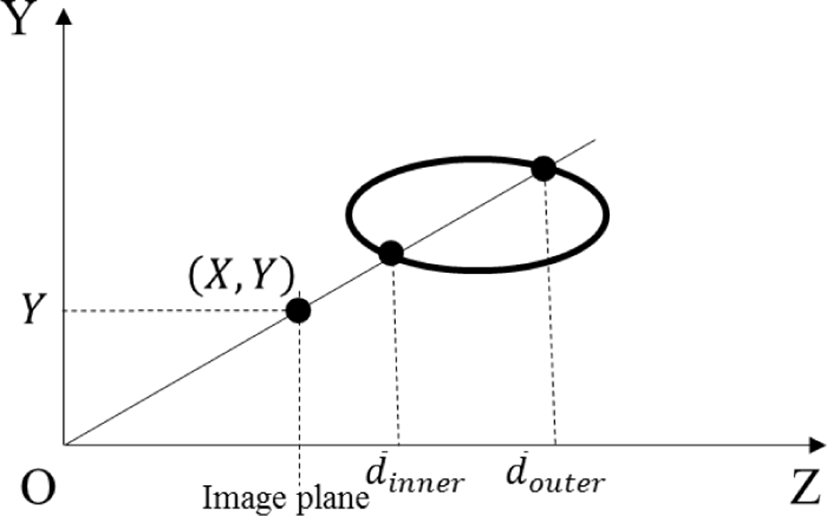Fig. 5. Predicted depth values in one ellipsoid.

Ellipsoid modeling is defined as finding the optimal parameters of the ellipsoid that minimize the prediction error. For ellipsoid modeling, the coordinates of the nose are set as the coordinate origin and the whole pixels of the face picture is subtracted from the depth pixel value of the nose. Then, the coordinates of the pixels substituted into Eq. (4) to obtain a following matrix equation:

$\begin{array}{l}AR=B\\ A=\left[\begin{array}{cc}{x}_{1}^{2}& {y}_{1}^{2}\\ {x}_{2}^{2}& {y}_{2}^{2}\\ ..& \cdots \end{array}\right]B=\left[\begin{array}{c}-\left(\frac{{d}_{1}^{2}}{{\delta }^{2}}+\frac{2{d}_{1}}{\delta }\right)\\ -\left(\frac{{d}_{2}^{2}}{{\delta }^{2}}+\frac{2{d}_{2}}{\delta }\right)\\ \cdots \end{array}\right]\\ R=\left[\begin{array}{c}1/{a}^{2}\\ 1/{b}^{2}\end{array}\right].\end{array}$
(7)

The ellipsoid parameters a and b are obtained by calculating R using the pseudo-inverse matrix of A by following equation:

$\begin{array}{l}R=B{A}^{+}\\ {A}^{+}={\left({A}^{\text{T}}A\right)}^{-1}{A}^{T}.\end{array}$
(8)

The proposed ellipsoid modeling method is applied to the depth picture shown in Fig. 6. In Fig. 6, distributions of the depth value of the body in the depth image is 800-1100.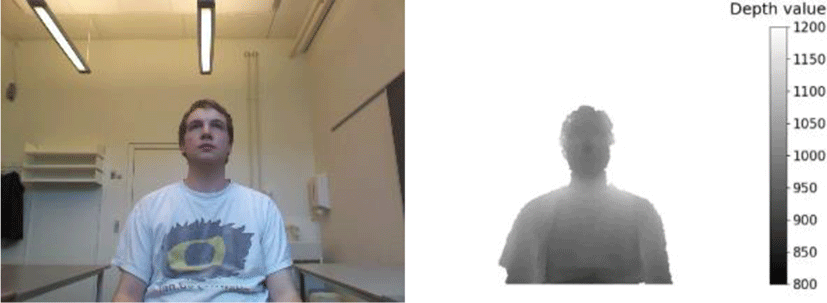Fig. 6. Depth picture with body.

Fig. 7 (b)-(c) show pictures of ellipsoid modeling from Fig. 7 (a) and differences between the captured and predicted pictures, respectively. This means that a size of the face depth picture can be reduced through ellipsoid modeling.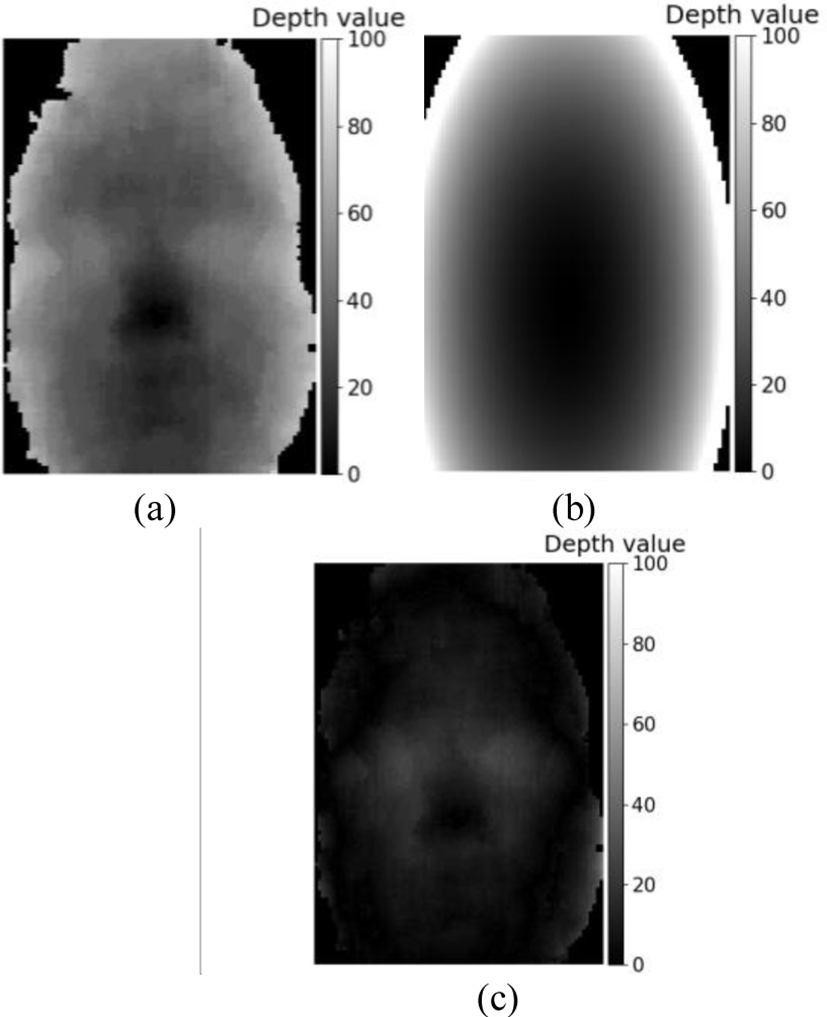Fig. 7. Prediction of face depth picture through ellipsoid modeling. (a) face depth picture, (b) predicted picture from ellipsoid modeling, and (c) Transmitted picture through ellipsoid modeling.

## III. SIMULATION RESULTS

In this paper, we measured the prediction accuracy of the depth face image using ellipsoid modeling. The parameters for nose detection is as follows: M and N are set to 5 and 15, respectively. The focal length f is 585.6.

We use a dataset  of face depth pictures for the simulation as shown in Fig. 8. The dataset for simulation is captured by Kinect and it includes 810 pictures. The depth pictures in the dataset are captured for 9 face poses of 30 people. Each pose is captured 3 times. In simulation pictures, pixel values that is not part of the face are removed.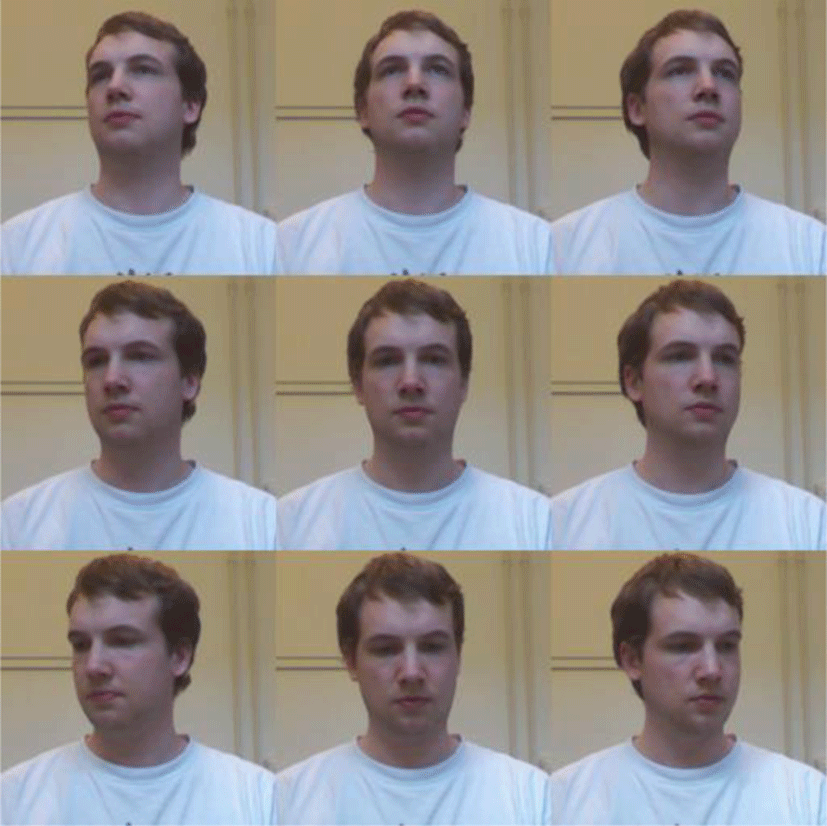Fig. 8. Face pictures with various face poses for simulation.

Measure nose detection accuracy. Because the depth face picture is accurately modeled through proposed method if a position of the nose is correctly found. Fig. 9 shows the success rate of finding the actual position of the nose. The nose is perfectly detected when the face pose is front, and the nose is detected with at least 85% accuracy even when the face pose is not front.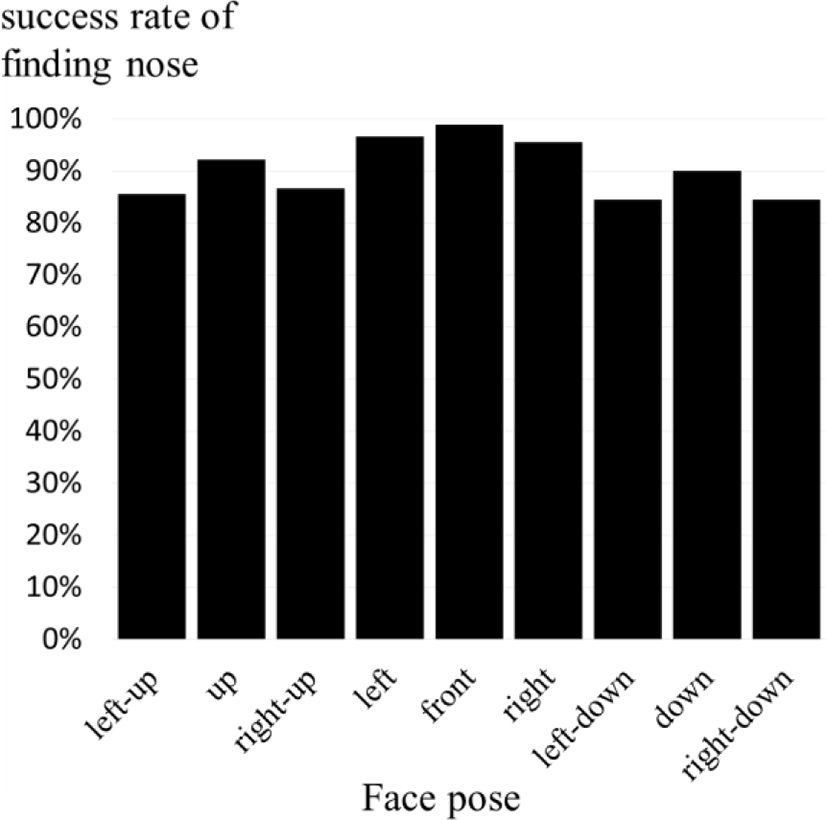Fig. 9. Success rate of finding actual position of nose.

The predicted accuracies by ellipsoid modeling are measured for each pose of the face. For measuring predicted accuracies through proposed ellipsoid modeling, we measure MSEs(Mean of Square Errors) between original and predicted pictures as follows:

$\text{MSE=}\frac{1}{w×h}\sum _{X=0}^{w}\sum _{Y=0}^{h}{\left(I\left(X,Y\right)-P\left(X,Y\right)\right)}^{2}$
(9)

where w and h mean a width and a height of the picture, respectively, and I(X, Y) and P(X, Y) mean pixel values at position (X, Y) of original and predicted pictures. The results are shown in Table 1. As the results, an average of MSE is measured to 20.3, and MSE is smallest when the face pose is the front.

Table 1. MSEs through ellipsoid modeling.
Face pose MSE
left-up 25.053
up 16.713
right-up 28.137
left 15.233
front 12.825
right 14.560
left-down 23.421
down 17.689
right-down 29.374

Fig. 10 shows a histogram of prediction errors between original and predicted pictures. In Fig. 10, an average of prediction errors is closed to 0, so this result shows that the proposed method effectively predicts the face depth pictures.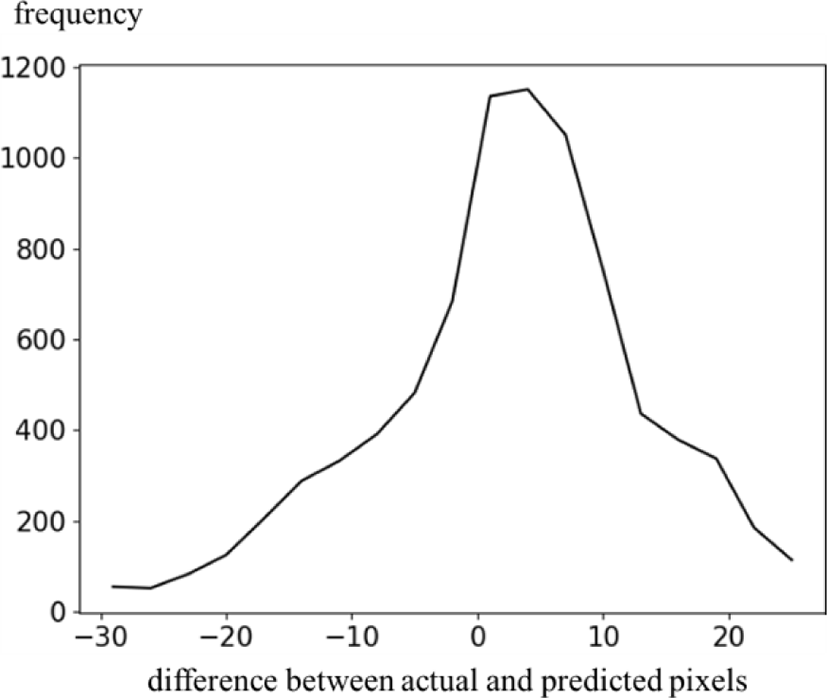Fig. 10. Histogram for prediction errors.

An entropy power for the difference picture between original and predicted pictures is investigated to predict approximate coding efficiency. The entropy power is defined as the output of white noise with the same frequency of all signals in an environment. The entropy power N(x) for the inputs x is calculated as follows:

$\begin{array}{ll}N\left(X\right)\hfill & =\frac{1}{2\pi e}{e}^{2h\left(X\right)}\hfill \\ h\left(X\right)\hfill & =-\sum {}_{i}{f}_{i}\mathrm{ln}\left({f}_{i}\right),\hfill \end{array}$
(10)

where fi is the probability of a signal i. In the results shown by Table 2. Table 2 shows that the face depth picture can be effectively compressed by the proposed method.

Table 2. Entropy power through ellipsoid modeling.
Face pose Entropy power
left-up 31.370
up 29.841
right-up 32.574
left 27.512
front 24.374
right 26.074
left-down 32.217
down 30.005
right-down 37.141

## IV. CONCLUSION

In this paper, we propose a method of predicting face picture through ellipsoid modeling to improve the transmission rates. The simulation results for the proposed method show that the face depth pictures can be efficiently predicted through the proposed method. In the face recognition methods using depth pictures that can accurately recognize the face without the influence of lighting, the proposed method is useful to quickly transmit the face depth pictures. The proposed method is expected to be applied to the field of identity recognition, which is recently increasing in importance.

## Acknowledgement

This research was supported by the BB21+ Project in 2019.

## REFERENCES

.

M. A. Turk and A. P. Pentland, “Face Recognition Using Eigenfaces,” in Proceeding of IEEE Computer Society Conference on Computer Vision and pattern Recognition, 586-591, 1991..

L. C. Paul and A. A. Suman, “Face Recognition Using Principal Component Analysis Method,” International Journal of Advanced Research in Computer Engineering & Technology, vol. 1, no. 9, pp. 135-139, 2012..

D. G. Lowe, “Object Recognition from Local Scale-invariant Features,” in Proceeding of the International Conference on Computer Vision, pp. 1150-1157, 1999..

C. Geng and X. Jiang, “Face Recognition Using SIFT Features,” in Proceeding of IEEE International Conference on Image Processing, pp. 3313-3316, 2009..

P. Viola, and M. J. Jones, “Rapid Object Detection Using A Boosted Cascade of Simple Features,” in Proceeding of IEEE Computer Society Conference on Computer Vision and Pattern Recognition, pp. 511-518, 2001..

S. K. Kwon, H. J. Kim, and D. S. Lee, D.S, “Face Recognition Method Based on Local Binary Pattern Using Depth Images,” Journal of the Korea Industrial Information Systems Research, vol. 22, no. 6, pp. 39-45, 2017..

S. K. Kwon, “Face Recognition Using Depth and Infrared Pictures,” in Proceeding of IEICE Nonlinear Theory and Its Applications, vol. 10, no. 1, pp. 2-15, 2019..

S. Grewatsch and E. Muller, “Evaluation of Motion Compensation and Coding Strategies for Compression of Depth Map Sequences,” in Proceeding of the Mathematics of Data/Image Coding, Compression, and Encryption VII, with Applications, pp. 117-125, 2004..

Y. Morvan, D. Farin, and P. H. N. deWith, “Depth-image Compression Based on An R-D Optimized Quadtree Decomposition for The Transmission of Multiview Images,” in Proceeding of the IEEE International Conference on Image Processing, pp. V105-V108, 2007..

S. Milani and G. Calvagno, “A Depth Image Coder Based on Progressive Silhouettes,” IEEE Signal Process. Letters, vol. 17, no. 8, pp. 711-714, 2010..

G. Shen, W. Kim, S. Narang, A. Orterga, J. Lee, and H. Wey, “Edge Adaptive Transform for Efficient Depth Map Coding,” in Proceeding of Picture Coding Symposium, pp. 566-569, 2010..

M. Maitre and M. Do, “Depth and Depth-Color Coding Using Shape Adaptive Wavelets,” Journal of Visual Communication and Image Representation, vol. 21 (5-6), pp. 513-522, 2010..

J. Fu, D. Miao, W. Yu, S. Wang, Y. Lu, and S. Li, “Kinect-Like Depth Data Compression,” IEEE Transactions on Multimedia, vol. 15, no. 6, pp. 1340-1352, 2013..

D. S. Lee and S. K. Kwon, “Intra Prediction of Depth Picture with Plane Modeling,” Symmetry, vol. 10, pp. 1–16, 2018..

S. K. Kwon, D. S. Lee, and Y. H. Park, “Depth Video Coding Method for Spherical Object,” Journal of the Korea Industrial Information Systems Research, vol. 21, no. 6, pp. 23-29, 2016..

R. I. Hg, P. Jasek, C. Rofidal, K. Nasrollahi, and T. B. Moeslund, “An RGB-D Database Using Microsoft’s Kinect for Windows for Face Detection,” in Proceeding of the IEEE 8th International Conference on Signal Image Technology & Internet Based Systems, pp. 42-46, 2012.## Authors

Dong-jin Park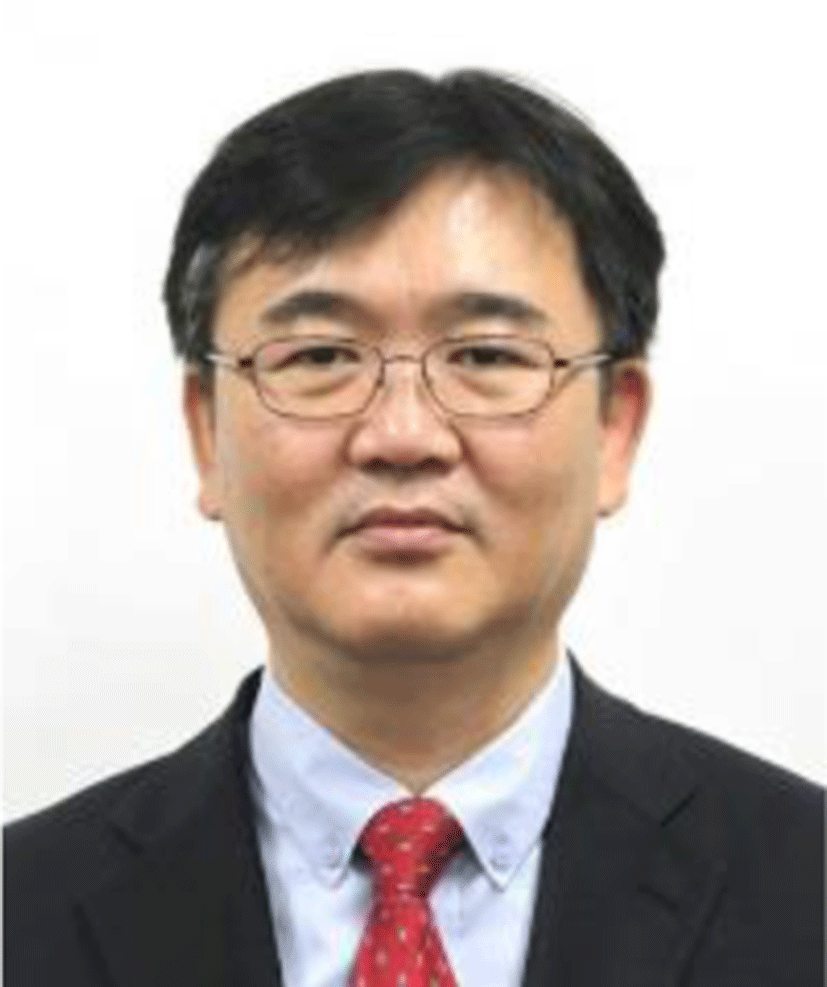Dong-jin Park (pktelpark@gmail.com) is currently a Ph.D. course in the Department of Computer Software Engineering at Dongeui University. He received the B.S. degree in Businesss Administration from Dong-A University in 1994 and the M.S. degree in the Department of Computer Software Engineering from Dongeui University in 2009. His research interest is in the areas of image processing and video processing.

Soon-Kak KwonSoon-Kak Kwon (skkwon@deu.ac.kr) received the B.S. degree in Electronic Engineering from Kyungpook National University, in 1990, the M.S. and Ph.D. degrees in Electrical Engineering from Korea Advanced Institute of Science and Technology (KAIST), in 1992 and 1998, respectively. From 1998 to 2000, he was a team manager at Technology Appraisal Center of Korea Technology Guarantee Fund. Since 2001, he has been a faculty member of Dongeui University, where he is now a professor in the Department of Computer Software Engineering. From 2003 to 2004, he was a visiting professor of the Department of Electrical Engineering in the University of Texas at Arlington. From 2010 to 2011, he was an international visiting research associate in the School of Engineering and Advanced Technology in Massey University. Prof. Kwon received the awards, Leading Engineers of the World 2008 and Foremost Engineers of the World 2008, from IBC, and best papers from Korea Multimedia Society, respectively. His biographical profile has been included in the 2008~2014, 2017~2019 Editions of Marquis Who’s Who in the World and the 2009/2010 Edition of IBC Outstanding 2000 Intellectuals of the 21st Century. His research interests are in the areas of image processing, video processing, and video transmission.